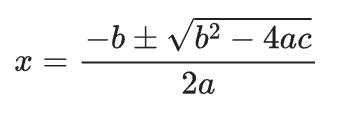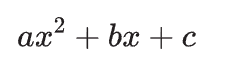SherpaQuestion

# Roots of a Quadratic Equation

6 months ago

·

13 Replies

·

501 views

R

Robert Richard

The derivative of the quadratic formula is both values ​​of x, which are obtained by addressing the quadratic equation. These derivatives of a quadratic equation are also called absolute nos of the formula. For example, the roots of the formula x2 - 3x - 4 = 0 are x = -1 and x = four because each satisfies the formula. that is,

At x = -1, (-1 )2 - 3( -1) - 4 = 1 + 3 - 4 = 0

At x = 4, (4 )2 - 3( 4) - 4 = 16 - 12 - 4 = 0

There are different methods for finding the derivative of a quadratic equation. The use of the quadratic formula calculator is one of them.Need help with your studies? Find a qualified and experienced tutor on Sherpa.

A
Ashish Gupta

Roots of quadratic equations are TWO values of `x ` ,If a quadratic equation can be factored, the roots of the equation can be found using the factors.

M
Margarita Donkoh Lockner

Yes, that's right! The use of the quadratic formula is one of the ways to find the roots of a quadratic equation. This formula is the following:where a, b and c are taken from:Hence, for your example, a=1, b=-3 and c=4.

Once you have put these in the quation, your answers for x are 4 and -1

A
Ashish Patel

The quadratic formula can be used to solve quadratic equations that you are unable to factorise. Using the formula (-b ± sqrt(b^2 - 4ac)) / 2a. Where a quadratic is in the form ax^2 + bx + c = 0

substituting the values of a=1 b=-3 and c=-4

x = (-(-3) ± √((-3)² - 4(1)(-4))) / (2(1))

when simplified results in

x = (3 ± √(9 + 16)) / 2

x = (3 ± √25) / 2

Hence

x = (3 + 5) / 2 or x = (3 - 5) / 2

x = -1/4

K
Kum Nagul Nirosh
1. You can factorize the multiple of ‘ac’ in a standard quadratic equation as ax2+bx+c=0. So you can rewrite the equation as x2-4x+x-4=0, now you can factorize as (x+1)(x-4)=0
2. You can also use standard quadratic solution equation as well which is,